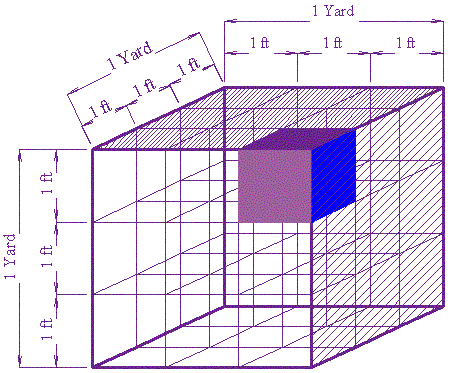# Conversion of Volume

Note: we also have Conversion Charts, and a Unit Converter.

First, you should understand Conversion of Length. If you are comfortable converting miles to kilometers, etc, then you are half way there.

## Volume is Length by Width by HeightTo convert Volume, remember that volume is length by width by height:

Volume = Length × Width ×  Height

So, we need to convert once for the length and once again for the width and once more for the height. It is that easy:

Just do the length conversion three times.

Here are some examples:

### Example: Convert 1 cubic yard into cubic feet.

Now, we know that three feet make a yard:

1 yd = 3 ft

So, the Length conversion is "multiply by 3".

And so the Volume conversion must be to multiply by 3 and multiply by 3 again and then multiply by 3 once again (ie once each for length, width and height)

The result is:

3 × 3 × 3 = 27

There are 27 cubic feet in a cubic yard.

Try to count the 1 ft cubes below, and you will see why:### Example: Convert 30 cubic feet into cubic meters (30 ft3 to m3)

The conversion for feet to meters is:

1 ft = 0.3048 m

So, the Length conversion is to multiply by 0.3048

And the Volume conversion must be to multiply by 0.3048, and multiply by 0.3048 and multiply by 0.3048 again:

30 × 0.3048 × 0.3048 × 0.3048 = 0.85

So, 30 ft3 = 0.85 m3